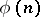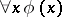# Carnap rule

(diff) ← Older revision | Latest revision (diff) | Newer revision → (diff)
rule of infinite induction,-rule
A derivation rule stating that if for an arithmetic formulathe propositionshave been proved, then the propositioncan be regarded as being proved. This rule was first brought into consideration by R. Carnap . Carnap's rule uses an infinite set of premises and is therefore inadmissible within the structure of the formal theories of D. Hilbert. The concept of a derivation in a system with the Carnap rule is undecidable. In mathematical logic one uses, for the study of formal arithmetic, the constructive Carnap rule: If there is an algorithm which for a natural numberprovides a derivation of the formula, then the propositioncan be regarded as being proved (the restricted-rule, the rule of constructive infinite induction). Classical arithmetic calculus, which by Gödel's theorem is incomplete, becomes complete on adding the constructive Carnap rule (see , ).